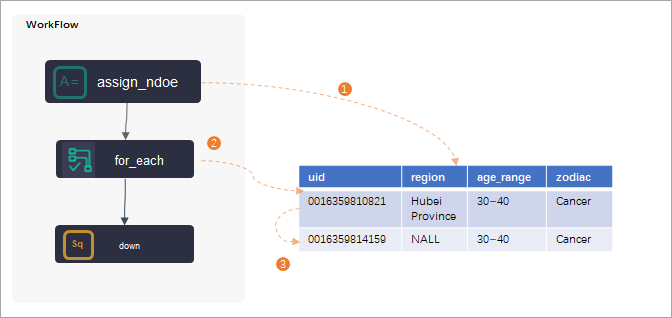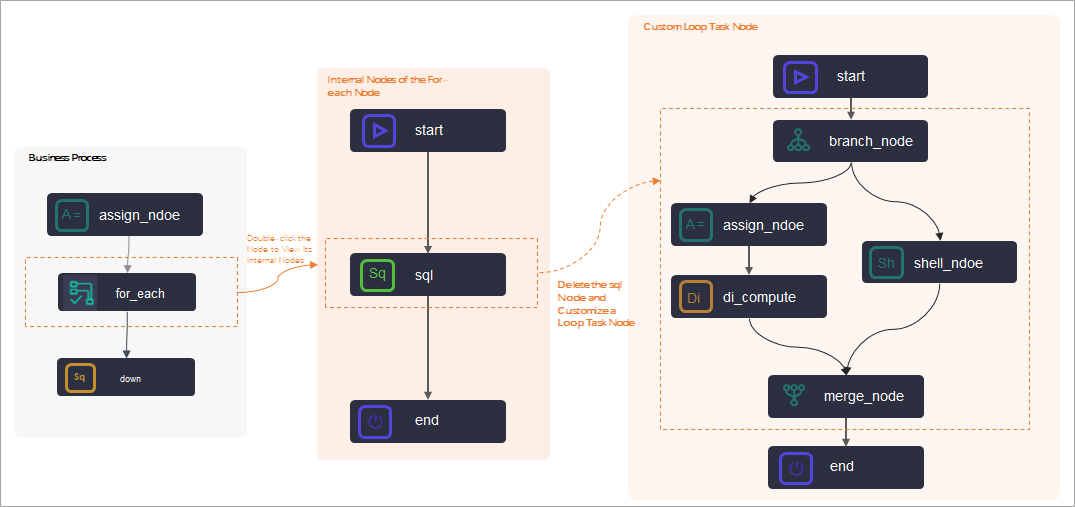DataWorks provides for-each nodes. You can use a for-each node to traverse the output of an assignment node in loops. You can also customize the workflow in a for-each node. This topic describes the composition and application logic of a for-each node.

Scenarios

In DataWorks, a for-each node is used to traverse the output of an assignment node in loops. Before you use a for-each node, you must configure the for-each node as a descendant node of an assignment node. After the assignment node passes its output to the for-each node, the for-each node traverses the output in loops.For more information about the limits of a for-each node, see Limits.
A for-each node contains inner nodes. The inner nodes are used to compile task code for traversal in loops. For more information, see Node composition.

Limits

• Dependencies

A for-each node is used to traverse the output of an assignment node in loops. You must configure the assignment node as an ancestor node of the for-each node.

• for-each nodes
• You can use for-each nodes only in DataWorks of the Standard Edition or a more advanced edition.
• A for-each node can traverse the output of an assignment node in a maximum of 128 loops. If the number of loops exceeds 128, an error is reported. The number of loops that are actually run by a for-each node varies based on the output of the assignment node that is configured as an ancestor node of the for-each node.
• If the output of an assignment node is a one-dimensional array, the number of loops is the number of elements in the array.

For example, a for-each node is configured as a descendant node of an assignment node in the Shell or Python 2 language, and the output of the assignment node is the one-dimensional array 2021-03-28,2021-03-29,2021-03-30,2021-03-31,2021-04-01. In this case, the for-each node traverses the output in five loops.

• If the output of an assignment node is a two-dimensional array, the number of loops is the number of rows in the array.
For example, a for-each node is configured as a descendant node of an assignment node in the ODPS SQL language, and the output of the assignment node is a two-dimensional array. The following code shows the two-dimensional array.
+---------------------------------------------------------------------------------+
| uid            | region         | age_range            | zodiac                 |
+---------------------------------------------------------------------------------+
| 0016359810821  | Hubei Province | 30 to 40 years old   | Cancer (constellation) |
| 0016359814159  | Unknown        | 30 to 40 years old   | Cancer (constellation) |
+---------------------------------------------------------------------------------+
In this case, the for-each node traverses the output in two loops.
• Inner nodes
• You can delete the existing dependencies between the inner nodes of a for-each node and customize the workflow in the for-each node. However, you must make sure that the workflow of the for-each node starts with the start node and ends with the end node.
• If you use a branch node as an inner node of a for-each node to perform logical judgments or traverse the output of an assignment node, you must also use a merge node.
• Testing
• If the workspace that you use is in standard mode, you cannot test a for-each node on the DataStudio page.

To test a for-each node and view the result, you must commit and deploy the for-each node to the production environment. Then, you can view the operational logs of the for-each node on the Operation Center page.

• To view the operational logs of a for-each node on the Operation Center page, right-click the for-each node in a directed acyclic graph (DAG) and select View Internal Nodes.

Node composition

In DataWorks, for-each nodes are a special type of node that contains inner nodes. After you create a for-each node, the following three inner nodes are created: start, sql, and end. The start node marks the start of a loop. The sql node runs a loop. The end node marks the end of a loop and determines whether to start the next loop. The three inner nodes are organized as a workflow to traverse the output of an assignment node.The preceding figure shows the inner nodes of a for-each node.
• sql node
By default, DataWorks creates an SQL node named sql in a for-each node. You can delete the sql node and customize inner nodes to run the loop task of the for-each node.
• If you want to use the sql node to run the loop task of your for-each node, double-click the node and write code on the configuration tab of the node.
• If the loop task of your for-each node is complex, you can create more inner nodes to run the task and connect the nodes based on your business requirements.
Note Before you customize inner nodes that are used to run the loop task of your for-each node, you can delete the dependencies between the original inner nodes and customize new inner nodes and a workflow in the for-each node. However, you must make sure that the workflow starts with the start node and ends with the end node.
• start and end nodes
The start node marks the start of a loop. The end node marks the end of a loop. The two nodes are not used to run loops.
Note The end node does not determine the number of loops. The number of loops that are actually run by a for-each node varies based on the output of the assignment node that is configured as an ancestor node of the for-each node.

Built-in variables

Each time you run a for-each node to traverse the output of an assignment node in loops, you can configure some built-in variables to obtain the number of loops that are run by the for-each node and the offset.
Built-in variable Description Comparison between a loop run by a for-each node and a for loop
\${dag.loopDataArray} The output of an assignment node. The value of this variable is equivalent to the code result in the for loop. Example:
data=[]
\${dag.foreach.current} The current data entry. Example of code in the for loop:
for(int i=0;i<data.length;i++) {
print(data[i]);
}
• data[i] is equivalent to \${dag.foreach.current}.
• i is equivalent to \${dag.offset}.
\${dag.offset} The offset of the current number of loops to 1.
\${dag.loopTimes} The current number of loops. -
If you understand the schema of your output table, you can configure the variables that are described in the following table to obtain the values of other variables.
Variable Description
\${dag.dag.foreach.current[n]} The data in a column of the current row in each loop if the output of an assignment node is a two-dimensional array.
\${dag.loopDataArray[i][j]} The data in Column j of Row i in the output if the output of an assignment node is a two-dimensional array.
\${dag.foreach.current[n]} The data in a specified column if the output of an assignment node is a one-dimensional array.

Examples of variable values

• Example 1
An assignment node in the Shell language is configured as an ancestor node of a for-each node, and the last output of the assignment node is 2021-03-28,2021-03-29,2021-03-30,2021-03-31,2021-04-01. The following table lists the values of the built-in variables in this example.
Note The output of the assignment node is a one-dimensional array. The array contains five elements. The elements are separated by commas (,). Therefore, the total number of loops that are run by the for-each node is 5.
Built-in variable Value obtained in the first loop Value obtained in the second loop
\${dag.loopDataArray} 2021-03-28,2021-03-29,2021-03-30,2021-03-31,2021-04-01
\${dag.foreach.current} 2021-03-28 2021-03-29
\${dag.offset} 0 1
\${dag.loopTimes} 1 2
\${dag.foreach.current} 2021-03-30
• Example 2
An assignment node in the ODPS SQL language is configured as an ancestor node of a for-each node. The following pieces of data are obtained after the last SELECT statement is executed:
+---------------------------------------------------------------------------------+
| uid            | region         | age_range            | zodiac                 |
+---------------------------------------------------------------------------------+
| 0016359810821  | Hubei Province | 30 to 40 years old   | Cancer (constellation) |
| 0016359814159  | Unknown        | 30 to 40 years old   | Cancer (constellation) |
+---------------------------------------------------------------------------------+
The following table lists the values of the built-in variables in this example.
Note The output of the assignment node is a two-dimensional array. The array contains two rows. Therefore, the total number of loops that are run by the for-each node is 2.
Built-in variable Value obtained in the first loop Value obtained in the second loop
\${dag.loopDataArray}
+---------------------------------------------------------------------------------+
| uid            | region         | age_range            | zodiac                 |
+---------------------------------------------------------------------------------+
| 0016359810821  | Hubei Province | 30 to 40 years old   | Cancer (constellation) |
| 0016359814159  | Unknown        | 30 to 40 years old   | Cancer (constellation) |
+---------------------------------------------------------------------------------+
\${dag.foreach.current} 0016359810821,Hubei Province,30 to 40 years old,Cancer (constellation) 0016359814159,Unknown,30 to 40 years old,Cancer (constellation)
\${dag.offset} 0 1
\${dag.loopTimes} 1 2
\${dag.dag.foreach.current} 0016359810821 0016359814159
\${dag.loopDataArray} 0016359814159